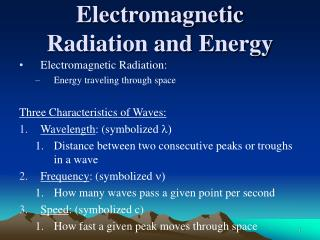DownloadDownload PresentationElectromagnetic Radiation and Energy

# Electromagnetic Radiation and Energy

Download Presentation## Electromagnetic Radiation and Energy

- - - - - - - - - - - - - - - - - - - - - - - - - - - E N D - - - - - - - - - - - - - - - - - - - - - - - - - - -
##### Presentation Transcript

1. Electromagnetic Radiation and Energy • Electromagnetic Radiation: • Energy traveling through space Three Characteristics of Waves: • Wavelength: (symbolized l) • Distance between two consecutive peaks or troughs in a wave • Frequency: (symbolized n) • How many waves pass a given point per second • Speed: (symbolized c) • How fast a given peak moves through space

2. Electromagnetic Radiation and Energy c = λ x ν c = speed of light = 2.9979 x 108 m/s ν = frequency (s-1 or Hz) λ = wavelength (m)

3. Spectra • Sunlight yields continuous spectrum • Energized gaseous elements yield certain wavelengths • Line emission spectrum

4. Rydberg • Why did gaseous atoms emit certain wavelengths? • Didn’t find out why, but came up with an equation • Rydberg equation • N=3, red line • N=4, green line • N=5, blue line • Lyman series • n > 1 to n = 1 • UV (invisible) • Balmer series • n > 2 to n = 2 • Visible wavelengths

5. The Bohr Model of the Atom • Explained Rydberg • Electron energy quantized • Electron only occupies certain energy levels or orbitals • If it didn’t, electron would crash into protons in nucleus • As “n” increases energy becomes less negative • Increases • Only certain amts of E may be absorbed/emitted • If electron in lowest possible energy level • Ground state • If electron in excited energy level • Excited state • One can calculate energy needed to raise H electron per atom from ground state (n=1) to first excited state (n=2)

6. Bohr’s Model • Explains emission spectrum of H • Movement of electrons from one quantized energy level to a lower one gave distinct emission wavelengths • Model only good for one electron system

7. Atomic orbital The probability function that defines the distribution of electron density in space around the atomic nucleus.

8. The s-orbital • The simplest orbital • The only orbital in the s-subshell • Occurs in every principal energy level • “s” stands for “sharp” • The first energy level only houses this orbital • Can house up to 2 electrons

9. The p-orbitals • Start in second principle energy level (n=2) • There are three p-orbitals in the p-subshell (see below) • And one s-orbital • “p” stands for “principle” • Can house up to 6 electrons • Has one nodal surface • Nodal plane = a planar surface in which there’s zero probability of find an electron 2px 2py 2pz

10. The d-orbitals • Start in third principle energy level (n=3) • There are five d-orbitals in the d-subshell • And one s-orbital • And three p-orbitals • Can house up to 10 electrons • “d” stands for “diffuse” • Has two nodal surfaces 3dyz 3dxz 3dxy 3dx2-y2 3dz2

11. The f-orbitals • Start in fourth principle energy level (n=4) • There are seven f-orbitals in the f-subshell • And one s-orbital • And three p-orbitals • And five d-orbitals • Can house up to 14 electrons • “f” stands for “fundamental” • Has 3 nodal surfaces

12. Electron configuration • Electron must be identified as to where it is located • Hydrogen: • One electron in first energy level and s-subshell • Thus, 1s1 (= Aufbau electron configuration) • 1 states energy level (n) • s designates subshell • Superscript 1 tells how many electrons are in the s-subshell • Can also use orbital box or line diagrams • Let’s take a look

13. Pauli Exclusion Principle • An atomic orbital holds a maximum of two electrons • Both electrons must have opposite spins • ms = +1/2 & -1/2

14. Hund’s Rule • Electron configuration most stable with electrons in half-filled orbitals before coupling

15. Subshell filling order – not what one expected

16. Using the Periodic Table to advantage

17. Short-hand vs. long-hand Aufbau electron configuration • F • Al • Ca • Br

18. Exercises • Give me the Aufbau electron configurations for: • Y • Te • Hf • Tl • 112

19. Sundry matters pertaining to d-block metals • Stability is increased when: • d-subshell is half-filled (d5) • d-subshell is completely filled (d10) • Electrons will be taken from the s-subshell to fill the d-subshell • But there is a limit • No more than 2 electrons taken from s-subshell • Given the above, which subshell electrons will d-block metals lose first when they ionize? • So what are the correct electron configurations of Cr and Ag? • Caveat • Not all metals follow the above; i.e., take from s-subshell and give to d-subshell • Ni & Pt, for example

20. Sundry matters pertaining to f-block metals • Stability is increased when: • f-subshell is half-filled (f7) • f-subshell is completely filled (f14) • Electron will be taken from the d-subshell to fill the f-subshell • Eu & Yb • Am & No